# 1.5   Input and Output

In this section we extend the set of simple abstractions (command-line input and standard output) that we have been using as the interface between our Java programs and the outside world to include standard input, standard drawing, and standard audio. Standard input makes it convenient for us to write programs that process arbitrary amounts of input and to interact with our programs; standard draw makes it possible for us to work with graphics; and standard audio adds sound.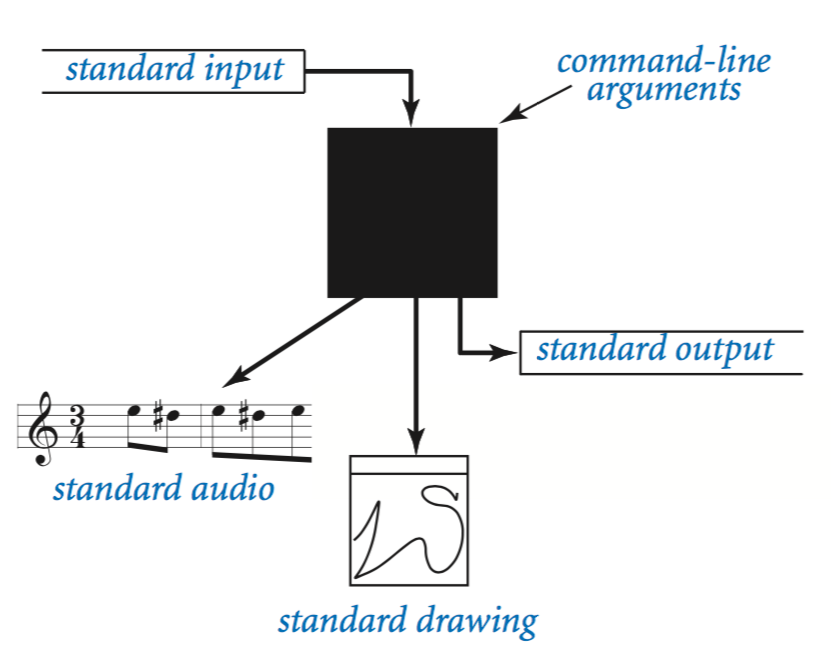## Bird's-eye view.

A Java program takes input values from the command line and prints a string of characters as output. By default, both command-line arguments and standard output are associated with an application that takes commands, which we refer to as the terminal window.
• Command-line arguments. All of our classes have a main() method that takes a String array args[] as argument. That array is the sequence of command-line arguments that we type. If we intend for an argument to be a number, we must use a method such as Integer.parseInt() to convert it from String to the appropriate type.
• Standard output. To print output values in our programs, we have been using System.out.println(). Java sends the results to an abstract stream of characters known as standard output. By default, the operating system connects standard output to the terminal window. All of the output in our programs so far has been appearing in the terminal window.

RandomSeq.java uses this model: It takes a command-line argument n and prints to standard output a sequence of n random numbers between 0 and 1.

To complete our programming model, we add the following libraries:

• Standard input. Read numbers and strings from the user.
• Standard drawing. Plot graphics.
• Standard audio. Create sound.

## Standard output.

Java's System.out.print() and System.out.println() methods implement the basic standard output abstraction that we need. Nevertheless, to treat standard input and standard output in a uniform manner (and to provide a few technical improvements), we use similar methods that are defined in our StdOut library: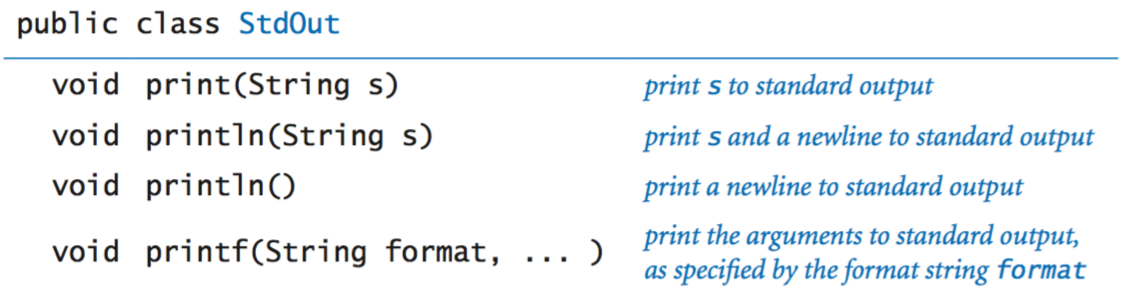Java's print() and println() methods are the ones that you have been using. The printf() method gives us more control over the appearance of the output.
• Formatted printing basics. In its simplest form, printf() takes two arguments. The first argument is called the format string. It contains a conversion specification that describes how the second argument is to be converted to a string for output.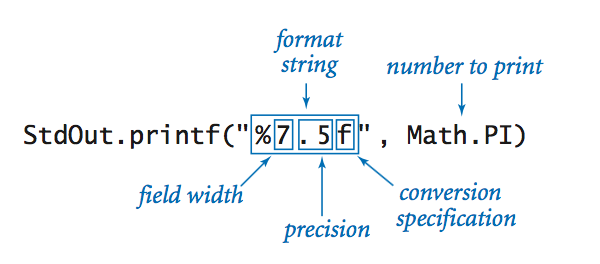Format strings begin with % and end with a one-letter conversion code. The following table summarizes the most frequently used codes: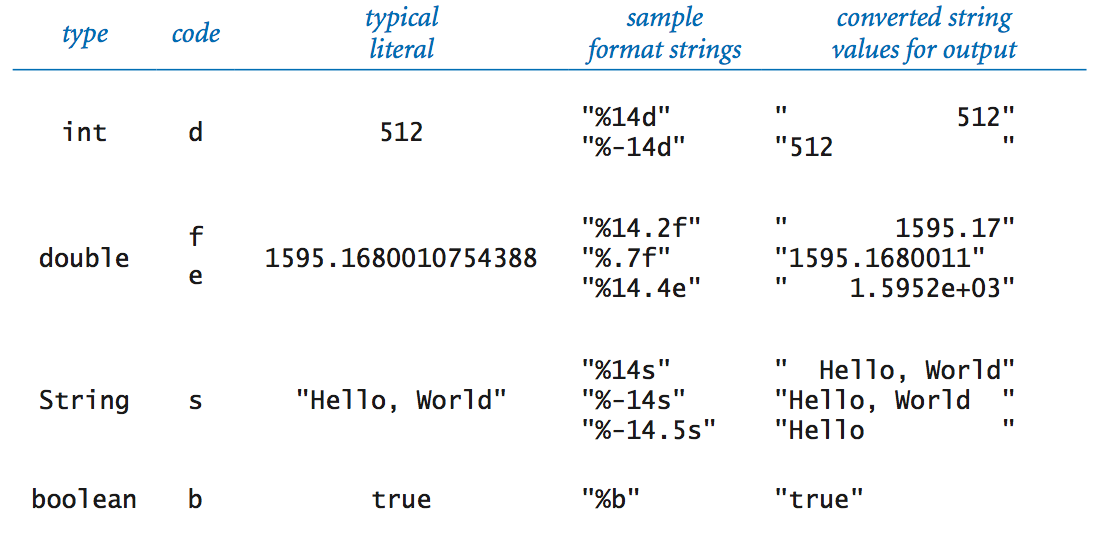• Format string. The format string can contain characters in addition to those for the conversion specification. The conversion specification is replaced by the argument value (converted to a string as specified) and all remaining characters are passed through to the output.
• Multiple arguments. The printf() function can take more than two arguments. In this case, the format string will have an additional conversion specification for each additional argument.
Here is more documentation on printf format string syntax.

## Standard input.

Our StdIn library takes data from a standard input stream that contains a sequence of values separated by whitespace. Each value is a string or a value from one of Java's primitive types. One of the key features of the standard input stream is that your program consumes values when it reads them. Once your program has read a value, it cannot back up and read it again. The library is defined by the following API: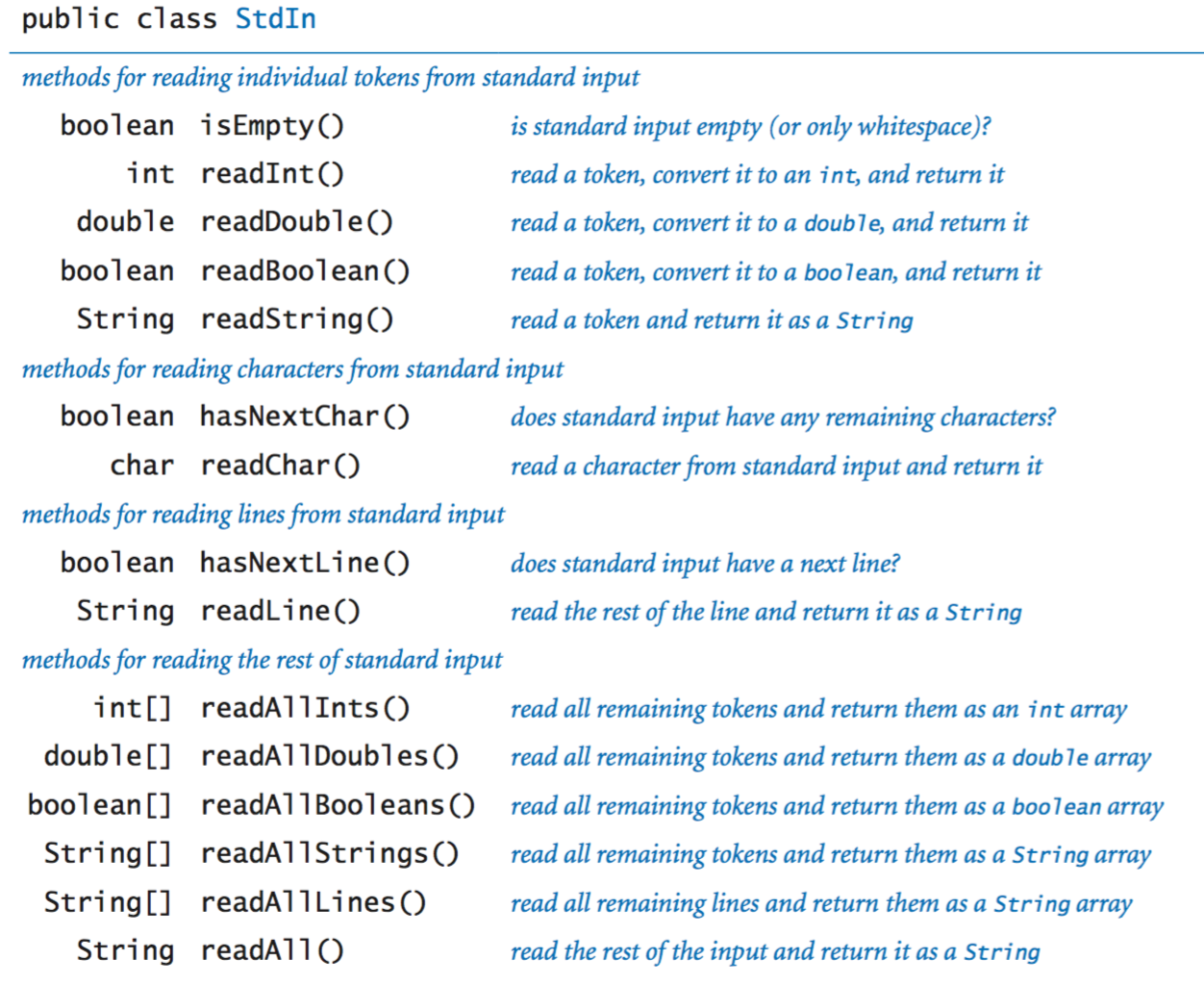We now consider several examples in detail.

• Typing input. When you use the java command to invoke a Java program from the command line, you actually are doing three things: (1) issuing a command to start executing your program, (2) specifying the values of the command-line arguments, and (3) beginning to define the standard input stream. The string of characters that you type in the terminal window after the command line is the standard input stream. For example, AddInts.java takes a command-line argument n, then reads n numbers from standard input and adds them, and prints the result to standard output:• Input format. If you type abc or 12.2 or true when StdIn.readInt() is expecting an int, then it will respond with an InputMismatchException. StdIn treats strings of consecutive whitespace characters as identical to one space and allows you to delimit your numbers with such strings.
• Interactive user input. TwentyQuestions.java is a simple example of a program that interacts with its user. The program generates a random integer and then gives clues to a user trying to guess the number. The fundamental difference between this program and others that we have written is that the user has the ability to change the control flow while the program is executing.
• Processing an arbitrary-size input stream. Typically, input streams are finite: your program marches through the input stream, consuming values until the stream is empty. But there is no restriction of the size of the input stream. Average.java reads in a sequence of real numbers from standard input and prints their average.

## Redirection and piping.

For many applications, typing input data as a standard input stream from the terminal window is untenable because doing so limits our program's processing power by the amount of data that we can type. Similarly, we often want to save the information printed on the standard output stream for later use. We can use operating system mechanisms to address both issues.
• Redirecting standard output to a file. By adding a simple directive to the command that invokes a program, we can redirect its standard output to a file, either for permanent storage or for input to some other program at a later time. For example, the command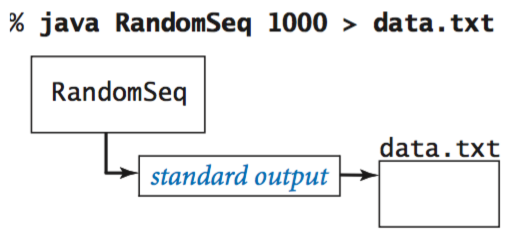specifies that the standard output stream is not to be printed in the terminal window, but instead is to be written to a text file named data.txt. Each call to StdOut.print() or StdOut.println() appends text at the end of that file. In this example, the end result is a file that contains 1,000 random values.
• Redirecting standard output from a file. Similarly, we can redirect standard input so that StdIn reads data from a file instead of the terminal window. For example, the command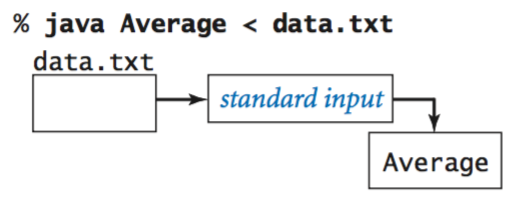reads a sequence of numbers from the file data.txt and computes their average value. Specifically, the < symbol is a directive to implement the standard input stream by reading from the file data.txt instead of by waiting for the user to type something into the terminal window. When the program calls StdIn.readDouble(), the operating system reads the value from the file. This facility to redirect standard input from a file enables us to process huge amounts of data from any source with our programs, limited only by the size of the files that we can store.
• Connecting two programs. The most flexible way to implement the standard input and standard output abstractions is to specify that they are implemented by our own programs! This mechanism is called piping. For example, the following command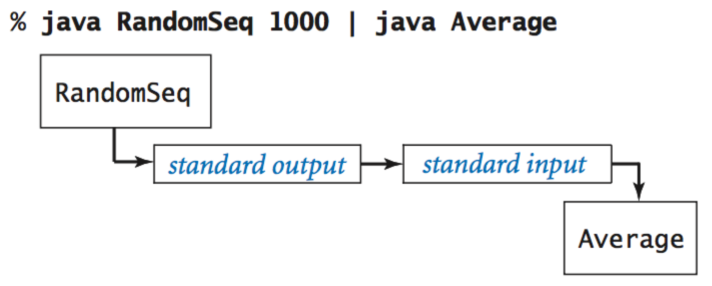specifies that the standard output for RandomSeq and the standard input stream for Average are the same stream.
• Filters. For many common tasks, it is convenient to think of each program as a filter that converts a standard input stream to a standard output stream in some way, RangeFilter.java takes two command-line arguments and prints on standard output those numbers from standard input that fall within the specified range.

Your operating system also provides a number of filters. For example, the sort filter puts the lines on standard input in sorted order:

 % java RandomSeq 5 | sort 0.035813305516568916 0.14306638757584322 0.348292877655532103 0.5761644592016527 0.9795908813988247 
Another useful filter is more, which reads data from standard input and displays it in your terminal window one screenful at a time. For example, if you type
 % java RandomSeq 1000 | more 
you will see as many numbers as fit in your terminal window, but more will wait for you to hit the space bar before displaying each succeeding screenful.

## Standard drawing.

Now we introduce a simple abstraction for producing drawings as output. We imagine an abstract drawing device capable of drawing lines and points on a two-dimensional canvas. The device is capable of responding to the commands that our programs issue in the form of calls to static methods in StdDraw. The primary interface consists of two kinds of methods: drawing commands that cause the device to take an action (such as drawing a line or drawing a point) and control commands that set parameters such as the pen size or the coordinate scales.
• Basic drawing commands. We first consider the drawing commands: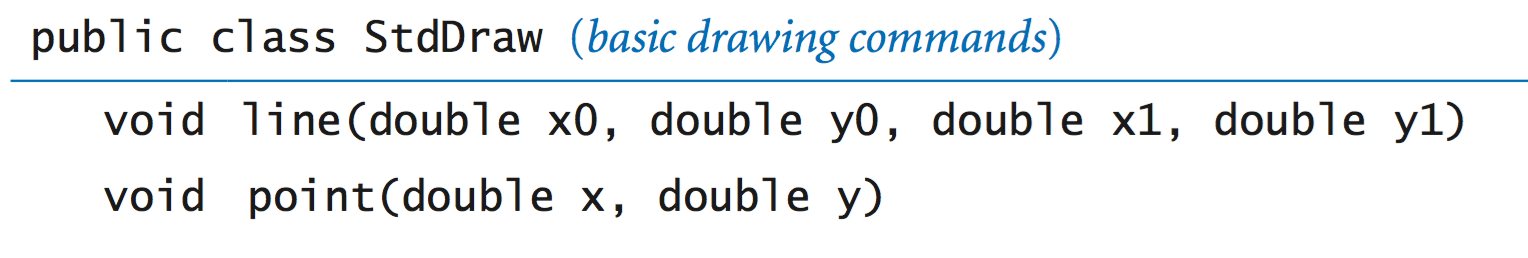These methods are nearly self-documenting: StdDraw.line(x0, y0, x1, y1) draws a straight line segment connecting the point (x0, y0) with the point (x1, y1). StdDraw.point(x, y) draws a spot centered on the point (x, y). The default coordinate scale is the unit square (all x- and y-coordinates between 0 and 1). The standard implementation displays the canvas in a window on your computer's screen, with black lines and points on a white background.

Your first drawing. The HelloWorld for graphics programming with StdDraw is to draw a triangle with a point inside. Triangle.java accomplishes this with three calls to StdDraw.line() and one call to StdDraw.point().

• Control commands. The default canvas size is 512-by-512 pixels and the default coordinate system is the unit square, but we often want to draw plots at different scales. Also, we often want to draw line segments of different thickness or points of different size from the standard. To accommodate these needs, StdDraw has the following methods: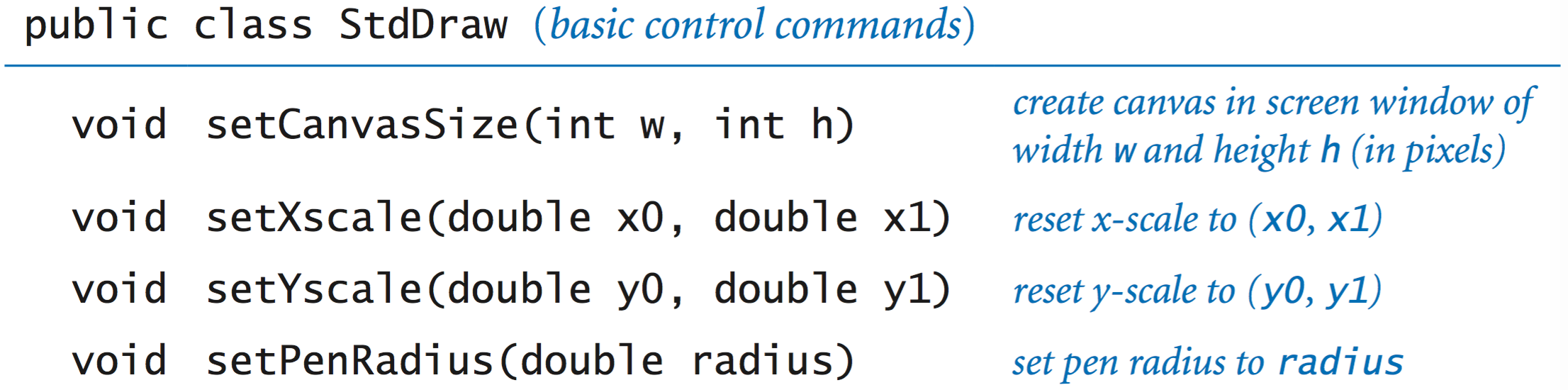For example, the two-call sequence
 StdDraw.setXscale(x0, x1); StdDraw.setYscale(y0, y1); 
sets the drawing coordinates to be within a bounding box whose lower-left corner is at (x0, y0) and whose upper-right corner is at (x1, y1).
• Filtering data to a standard drawing. PlotFilter.java reads a sequence of points defined by (x, y) coordinates from standard input and draws a spot at each point. It adopts the convention that the first four numbers on standard input specify the bounding box, so that it can scale the plot.

% java PlotFilter < USA.txt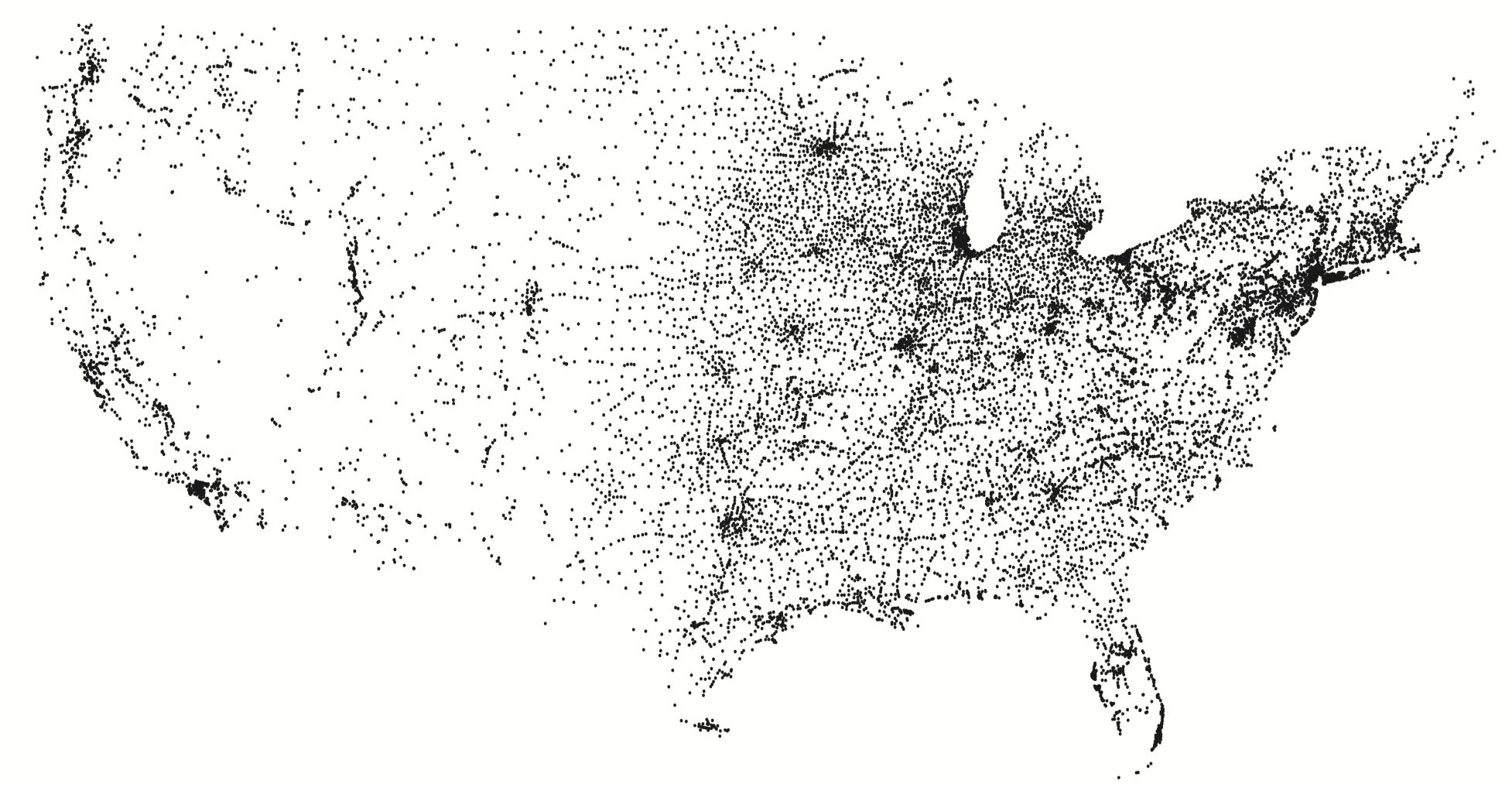• Plotting a function graph. FunctionGraph.java plots the function y = sin(4x) + sin(20x) in the interval (0, π). There are an infinite number of points in the interval, so we have to make do with evaluating the function at a finite number of points within the interval. We sample the function by choosing a set of x-values, then computing y-values by evaluating the function at each x-value. Plotting the function by connecting successive points with lines produces what is known as a piecewise linear approximation.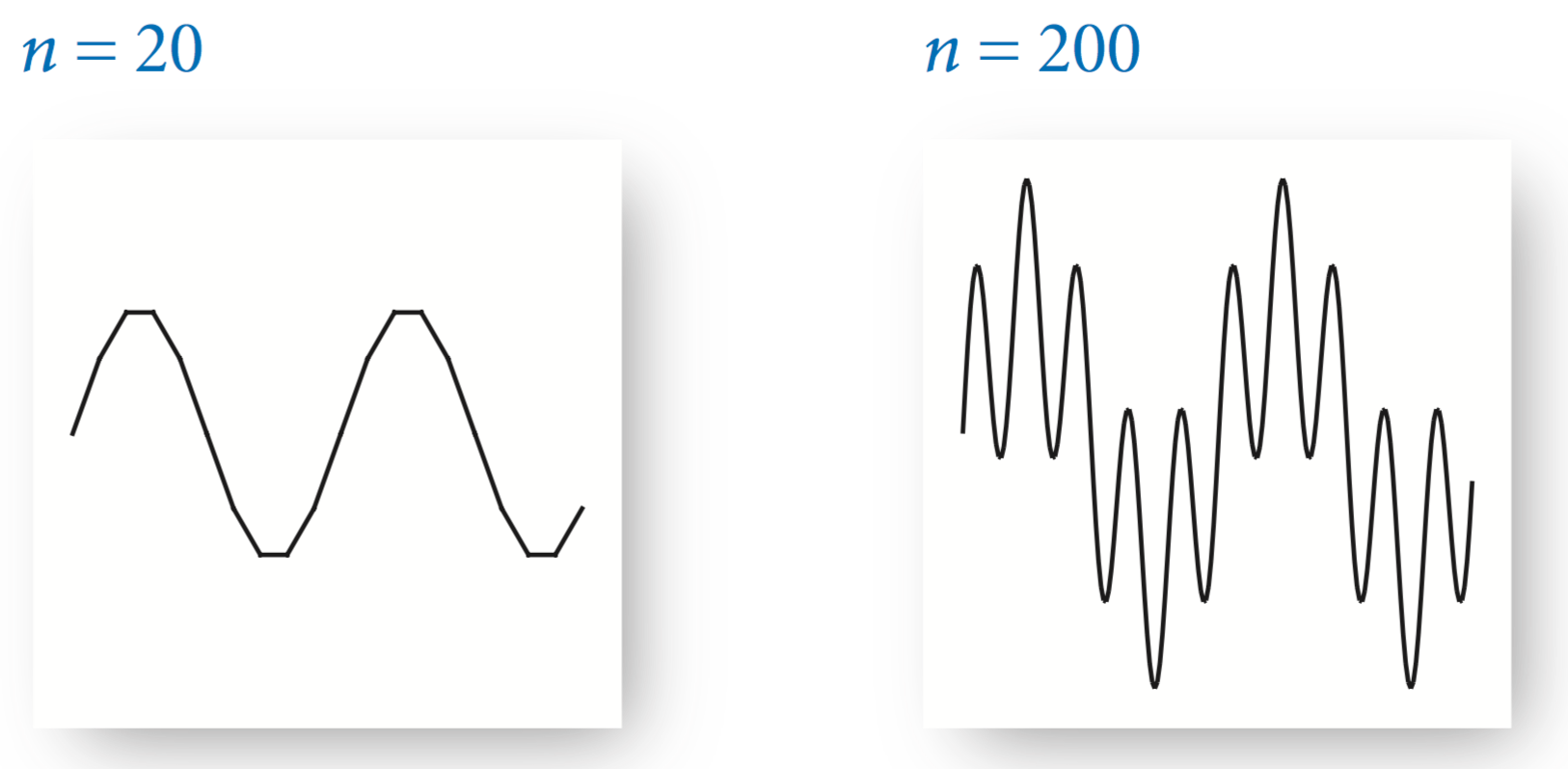• Outline and filled shapes. StdDraw also includes methods to draw circles, rectangles, and arbitrary polygons. Each shape defines an outline. When the method name is just the shape name, that outline is traced by the drawing pen. When the method name begins with filled, the named shape is instead filled solid, not traced.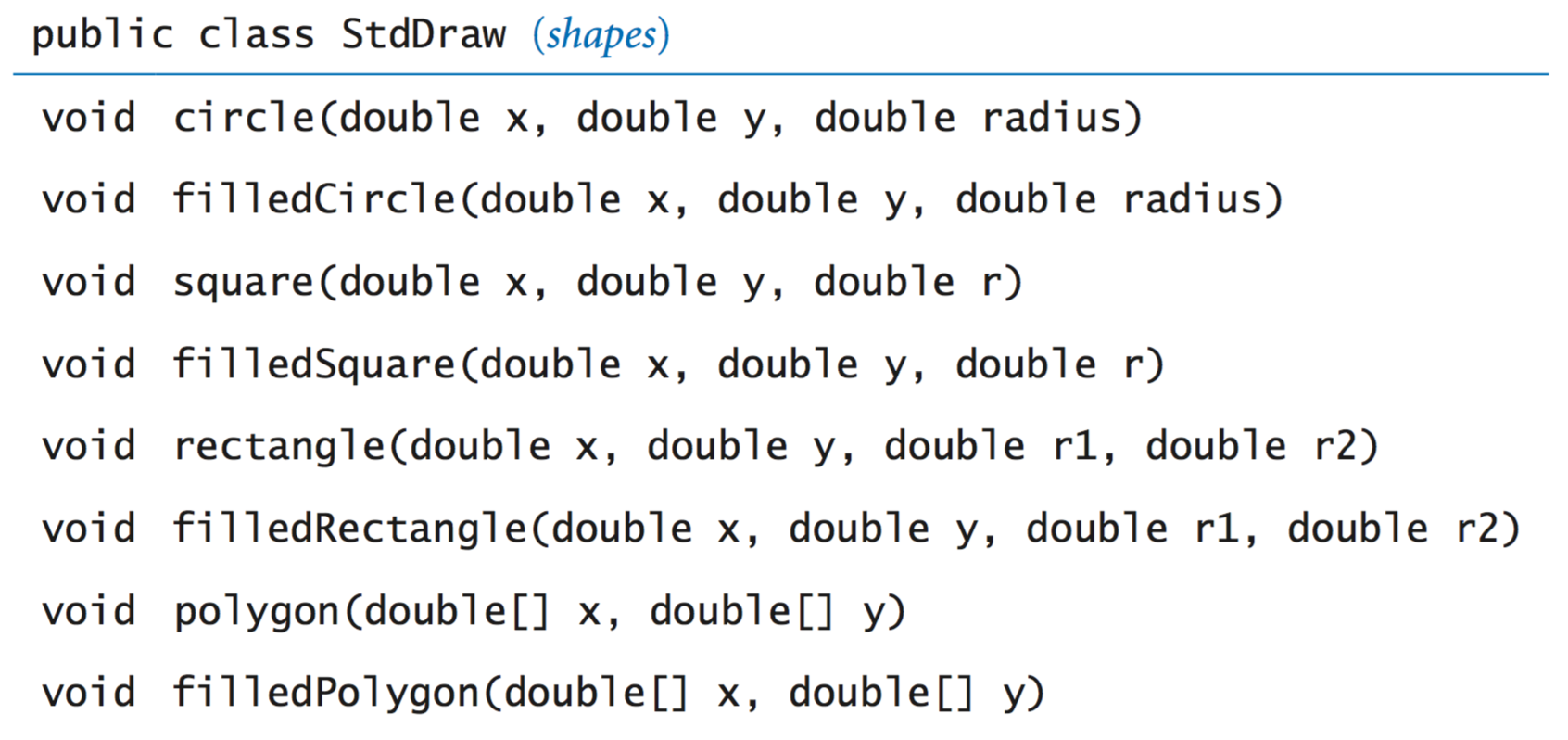The arguments for circle() define a circle of radius r; the arguments for square() define a square of side length 2r centered on the given point; and the arguments for polygon() define a sequence of points that we connect by lines, including one from the last point to the first point.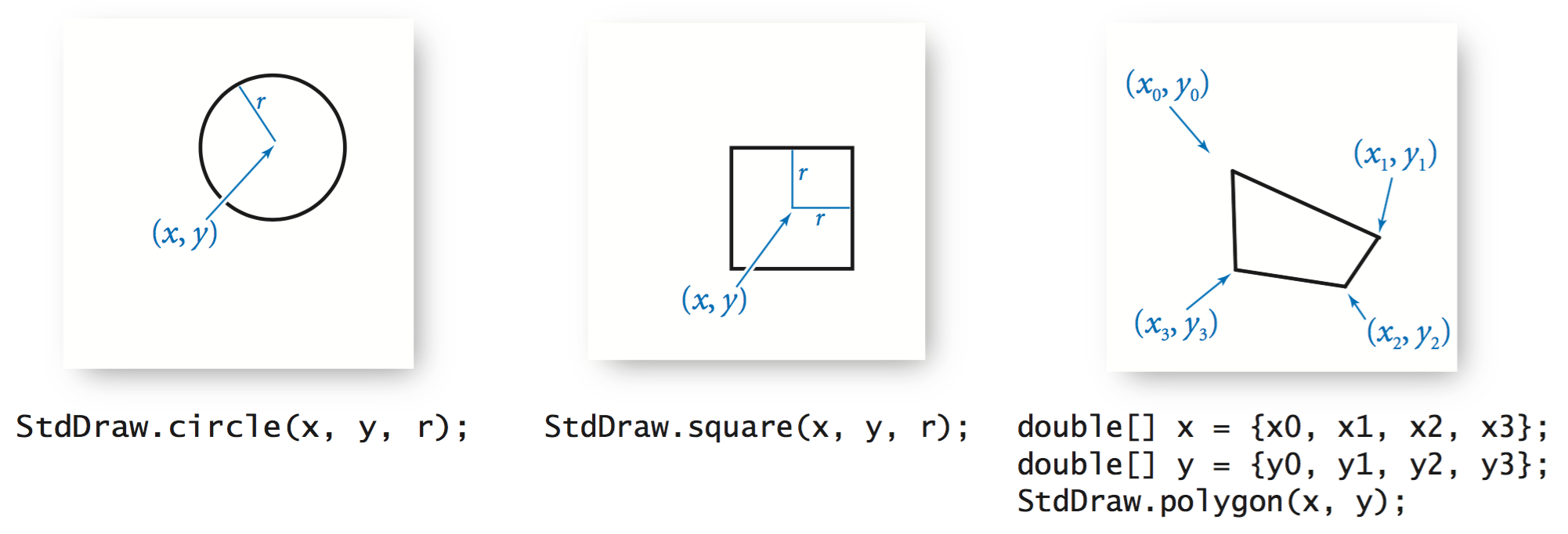• Text and color. To annotate or highlight various elements in your drawings, StdDraw includes methods for drawing text, setting the font, and setting the the ink in the pen.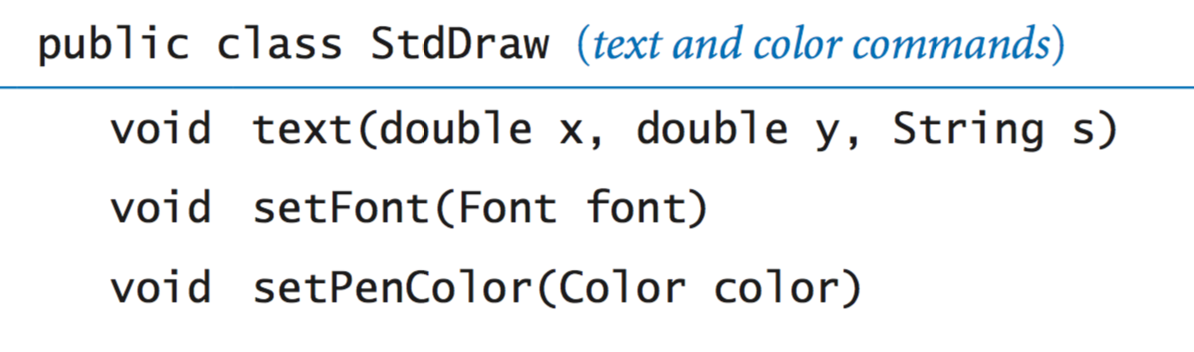In this code, java.awt.Font and java.awt.Color are abstractions that are implemented with non-primitive types that you will learn about in Section 3.1. Until then, we leave the details to StdDraw. The default ink color is black; the default font is a 16-point plain Serif font.
• Double buffering. StdDraw supports a powerful computer graphics feature known as double buffering. When double buffering is enabled by calling enableDoubleBuffering(), all drawing takes place on the offscreen canvas. The offscreen canvas is not displayed; it exists only in computer memory. Only when you call show() does your drawing get copied from the offscreen canvas to the onscreen canvas, where it is displayed in the standard drawing window. You can think of double buffering as collecting all of the lines, points, shapes, and text that you tell it to draw, and then drawing them all simultaneously, upon request. One reason to use double buffering is for efficiency when performing a large number of drawing commands.
• Computer animations. Our most important use of double buffering is to produce computer animations, where we create the illusion of motion by rapidly displaying static drawings. We can produce animations by repeating the following four steps:
• Clear the offscreen canvas.
• Draw objects on the offscreen
• Copy the offscreen canvas to the onscreen canvas.
• Wait for a short while.

In support of these steps, the StdDraw has several methods: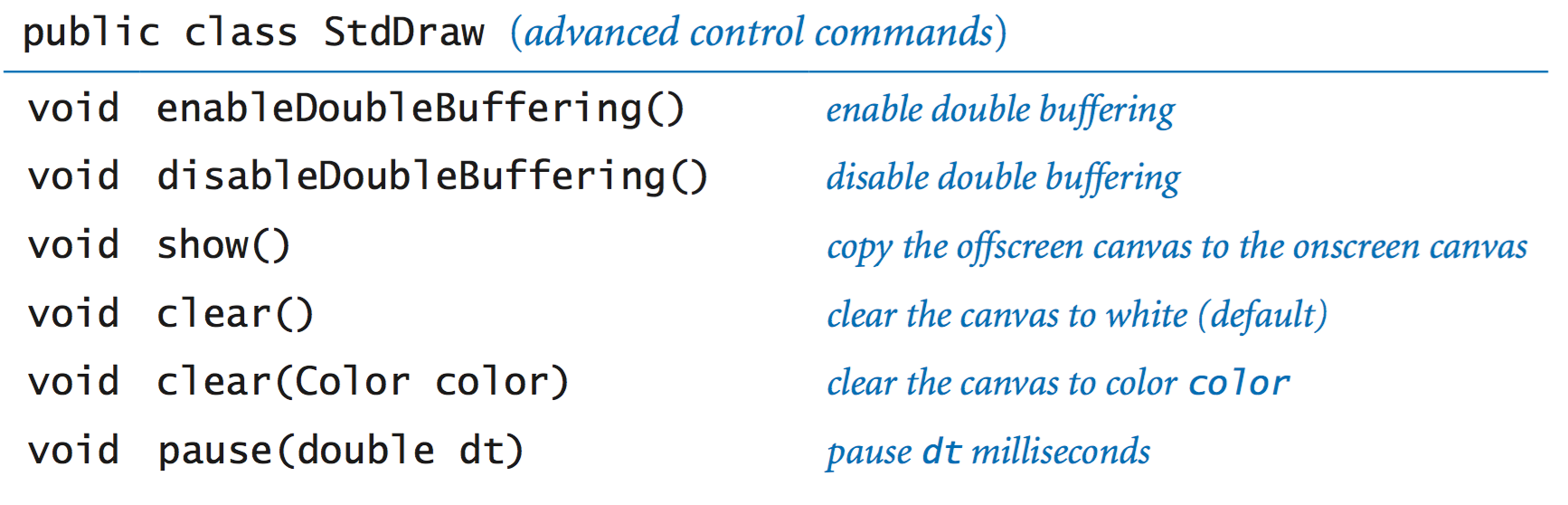The "Hello, World" program for animation is to produce a black ball that appears to move around on the canvas, bouncing off the boundary according to the laws of elastic collision. Suppose that the ball is at position (x, y) and we want to create the impression of having it move to a new position, say (x + 0.01, y + 0.02). We do so in four steps:

• Clear the offscreen canvas to white.
• Draw a black ball at the new position on the offscreen canvas.
• Copy the offscreen canvas to the onscreen canvas.
• Wait for a short while.

To create the illusion of movement, BouncingBall.java iterates these steps for a whole sequence of positions of the ball.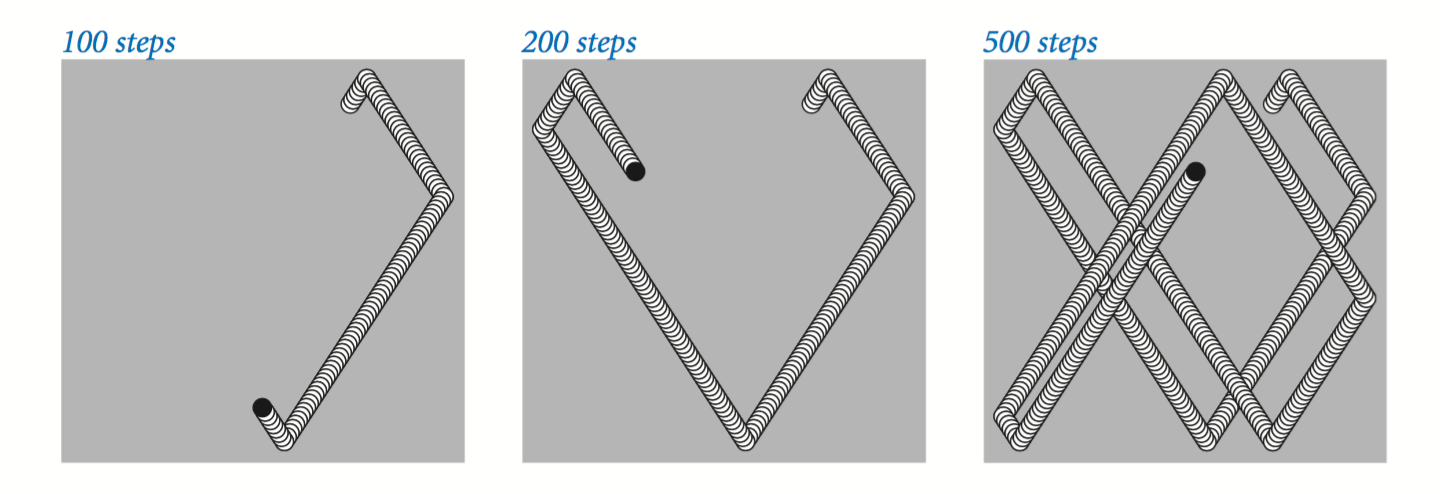• Images. Our standard draw library supports drawing pictures as well as geometric shapes. The command StdDraw.picture(x, y, filename) plots the image in the given filename (either JPEG, GIF, or PNG format) on the canvas, centered on (x, y). BouncingBallDeluxe.java illustrates an example where the bouncing ball is replaced by an image of a tennis ball.
• User interaction. Our standard draw library also includes methods so that the user can interact with the window using the mouse.

 double mouseX() return x-coordinate of mouse double mouseY() return y-coordinate of mouse boolean mousePressed() is the mouse currently being pressed? 
• A first example. MouseFollower.java is the HelloWorld of mouse interaction. It draws a blue ball, centered on the location of the mouse. When the user holds down the mouse button, the ball changes color from blue to cyan.
• A simple attractor. OneSimpleAttractor.java simulates the motion of a blue ball that is attracted to the mouse. It also accounts for a drag force.
• Many simple attractors. SimpleAttractors.java simulates the motion of 20 blue balls that are attracted to the mouse. It also accounts for a drag force. When the user clicks, the balls disperse randomly.
• Springs. Springs.java implements a spring system.

## Standard audio.

StdAudio is a library that you can use to play and manipulate sound files. It allows you to play, manipulate and synthesize sound.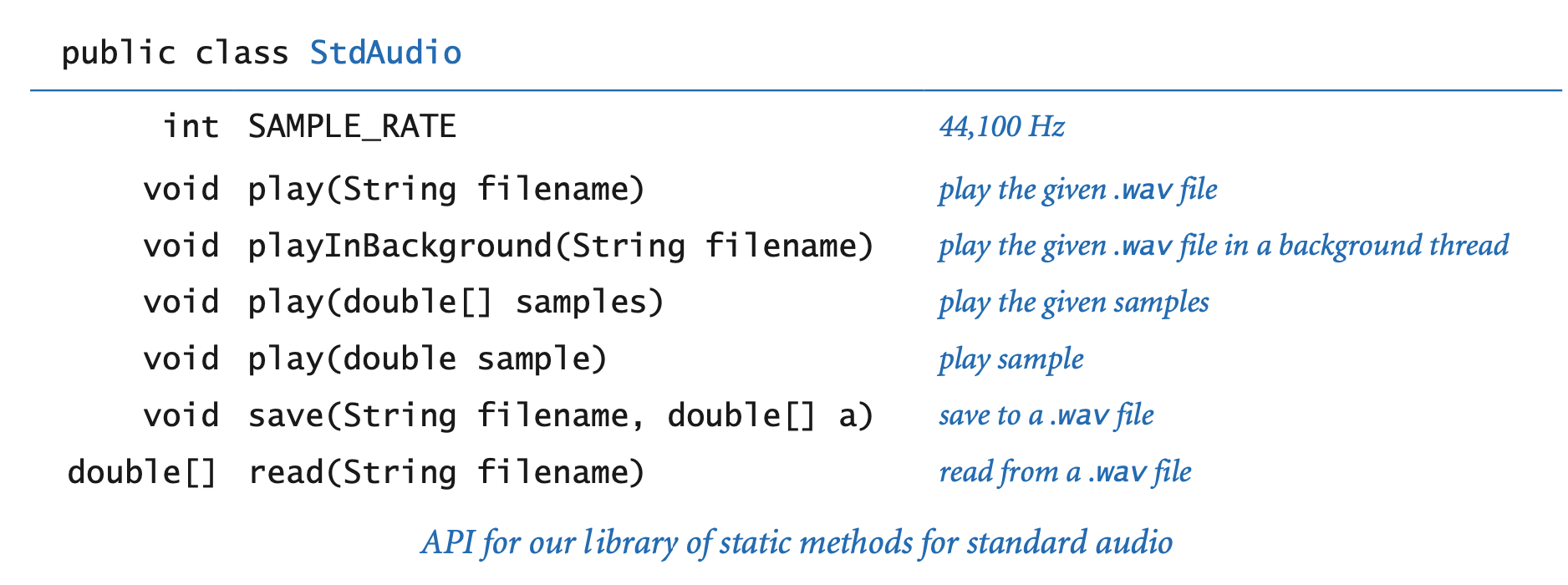We introduce some some basic concepts behind one of the oldest and most important areas of computer science and scientific computing: digital signal processing.
• Concert A. Concert A is a sine wave, scaled to oscillate at a frequency of 440 times per second. The function sin(t) repeats itself once every 2π units on the x-axis, so if we measure t in seconds and plot the function sin(2πt × 440) we get a curve that oscillates 440 times per second. The amplitude (y-value) corresponds to the volume. We assume it is scaled to be between −1 and +1.
• Other notes. A simple mathematical formula characterizes the other notes on the chromatic scale. They are divided equally on a logarithmic (base 2) scale: there are twelve notes on the chromatic scale, and we get the ith note above a given note by multiplying its frequency by the (i/12)th power of 2.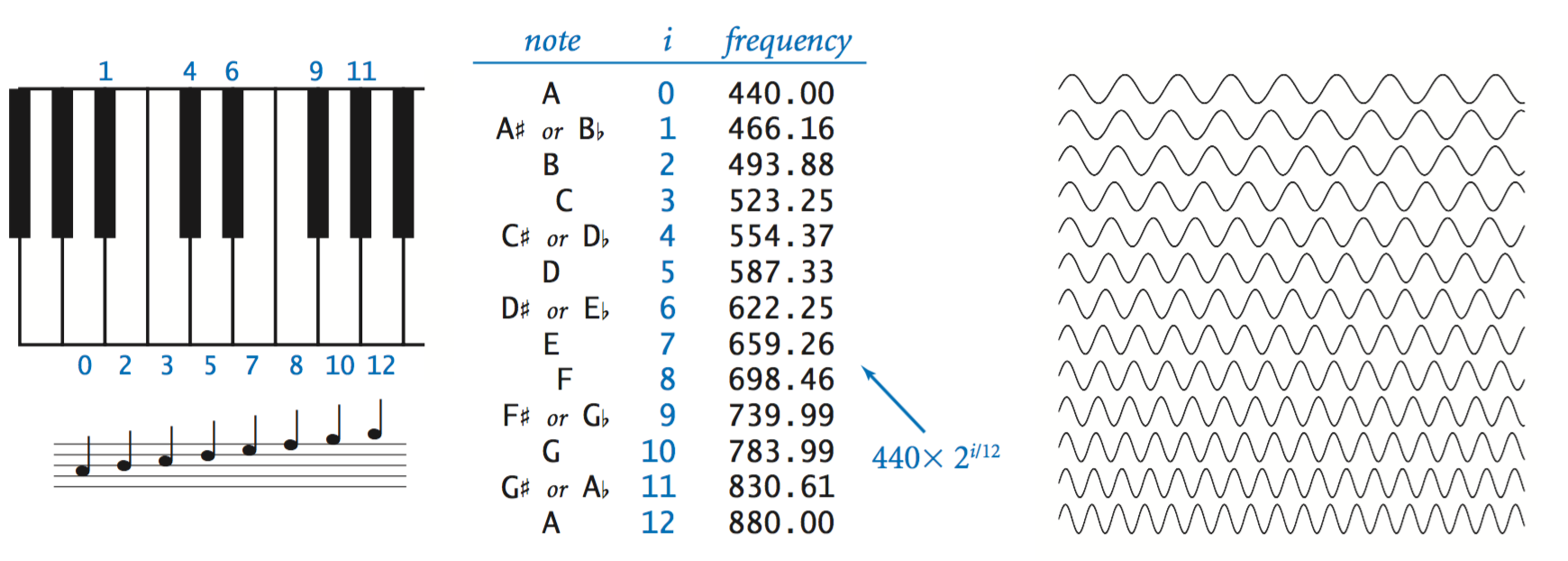When you double or halve the frequency, you move up or down an octave on the scale. For example 880 hertz is one octave above concert A and 110 hertz is two octaves below concert A.
• Sampling. For digital sound, we represent a curve by sampling it at regular intervals, in precisely the same manner as when we plot function graphs. We sample sufficiently often that we have an accurate representation of the curve—a widely used sampling rate is 44,100 samples per second. It is that simple: we represent sound as an array of numbers (real numbers that are between −1 and +1).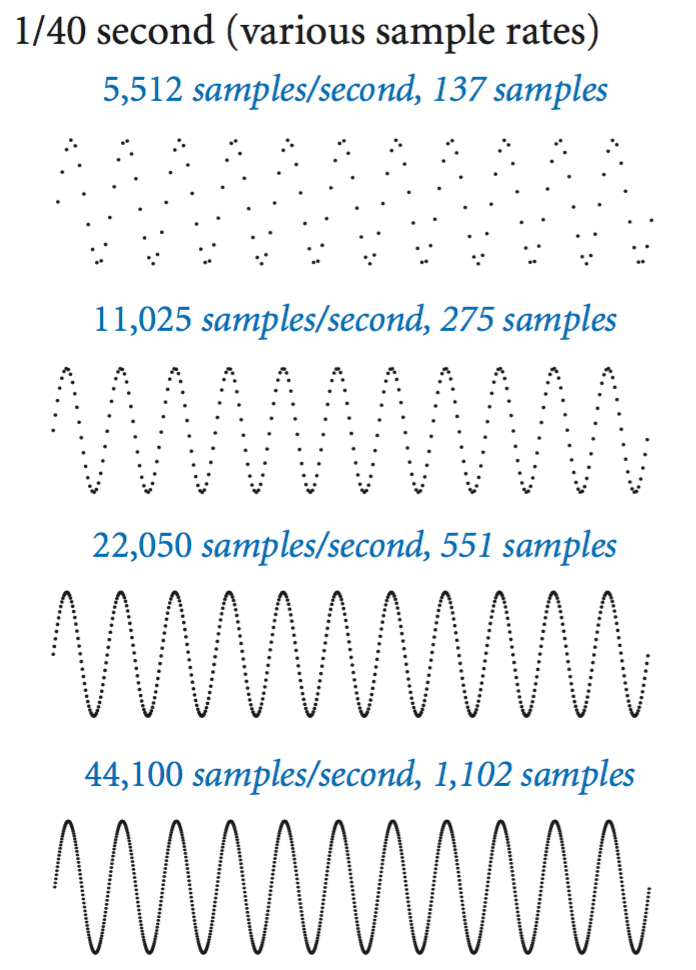For example, the following code fragment plays concert A for 10 seconds.
 int SAMPLING_RATE = 44100; double hz = 440.0; double duration = 10.0; int n = (int) (SAMPLING_RATE * duration); double[] a = new double[n+1]; for (int i = 0; i <= n; i++) { a[i] = Math.sin(2 * Math.PI * i * hz / SAMPLING_RATE); } StdAudio.play(a); 
• Play that tune. PlayThatTune.java is an example that shows how easily we can create music with StdAudio. It takes notes from standard input, indexed on the chromatic scale from concert A, and plays them on standard audio.

#### Exercises

1. Write a program MaxMin.java that reads in integers (as many as the user enters) from standard input and prints out the maximum and minimum values.
2. Write a program Stats.java that takes an integer command-line argument n, reads n floating-point numbers from standard input, and prints their mean (average value) and sample standard deviation (square root of the sum of the squares of their differences from the average, divided by n−1).
3. Write a program LongestRun.java that reads in a sequence of integers and prints out both the integer that appears in a longest consecutive run and the length of the run. For example, if the input is 1 2 2 1 5 1 1 7 7 7 7 1 1, then your program should print Longest run: 4 consecutive 7s.
4. Write a program WordCount.java that reads in text from standard input and prints out the number of words in the text. For the purpose of this exercise, a word is a sequence of non-whitespace characters that is surrounded by whitespace.
5. Write a program Closest.java that takes three floating-point command-line arguments $$x, y, z$$, reads from standard input a sequence of point coordinates $$(x_i, y_i, z_i)$$, and prints the coordinates of the point closest to $$(x, y, z)$$. Recall that the square of the distance between $$(x, y, z)$$ and $$(x_i, y_i, z_i)$$ is $$(x - x_i)^2 + (y - y_i)^2 + (z - z_i)^2$$. For efficiency, do not use Math.sqrt() or Math.pow().
6. Given the positions and masses of a sequence of objects, write a program to compute their center-of-mass or centroid. The centroid is the average position of the n objects, weighted by mass. If the positions and masses are given by (xi, yi, mi), then the centroid (x, y, m) is given by:
 m = m1 + m2 + ... + mn x = (m1x1 + ... + mnxn) / m y = (m1y1 + ... + mnyn) / m 

Write a program Centroid.java that reads in a sequence of positions and masses (xi, yi, mi) from standard input and prints out their center of mass (x, y, m). Hint: model your program after Average.java.

7. Write a program Checkerboard.java that takes a command-line argument n and plots an n-by-n checkerboard with red and black squares. Color the lower-left square red.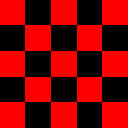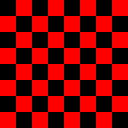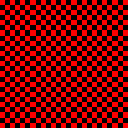8. Write a program Rose.java that takes a command-line argument n and plots a rose with n petals (if n is odd) or 2n petals (if n is even) by plotting the polar coordinates (r, θ) of the function r = sin(n × θ) for θ ranging from 0 to 2π radians. Below is the desired output for n = 4, 7, and 8.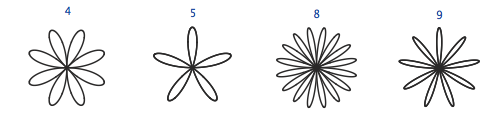9. Write a program Banner.java that takes a string s from the command line and display it in banner style on the screen, moving from left to right and wrapping back to the beginning of the string as the end is reached. Add a second command-line argument to control the speed.
10. Write a program Circles.java that draws filled circles of random size at random positions in the unit square, producing images like those below. Your program should take four command-line arguments: the number of circles, the probability that each circle is black, the minimum radius, and the maximum radius.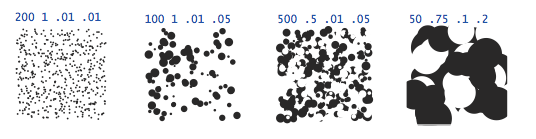#### Creative Exercises

1. Spirographs. Write a program Spirograph.java that takes three command-line arguments R, r, and a and draws the resulting spirograph. A spirograph (technically, an epicycloid) is a curve formed by rolling a circle of radius r around a larger fixed circle or radius R. If the pen offset from the center of the rolling circle is (r+a), then the equation of the resulting curve at time t is given by
 x(t) = (R+r)*cos(t) - (r+a)*cos(((R+r)/r)*t) y(t) = (R+r)*sin(t) - (r+a)*sin(((R+r)/r)*t) 

Such curves were popularized by a best-selling toy that contains discs with gear teeth on the edges and small holes that you could put a pen in to trace spirographs.

For a dramatic 3d effect, draw a circular image, e.g., earth.gif instead of a dot, and show it rotating over time. Here's a picture of the resulting spirograph when R = 180, r = 40, and a = 15.

2. Clock. Write a program Clock.java that displays an animation of the second, minute, and hour hands of an analog clock. Use the method StdDraw.show(1000) to update the display roughly once per second.

Hint: this may be one of the rare times when you want to use the % operator with a double - it works the way you would expect.

3. Oscilloscope. Write a program Oscilloscope.java to simulate the output of an oscilloscope and produce Lissajous patterns. These patterns are named after the French physicist, Jules A. Lissajous, who studied the patterns that arise when two mutually perpendicular periodic disturbances occur simultaneously. Assume that the inputs are sinusoidal, so tha the following parametric equations describe the curve:
 x = Ax sin (wxt + θx) y = Ay sin (wyt + θy) Ax, Ay = amplitudes wx, wy = angular velocity θx, θy = phase factors 

Take the six parameters Ax, wx, θx, θy, wy, and θy from the command line.

For example, the first image below has Ax = Ay = 1, wx = 2, wy = 3, θx = 20 degrees, θy = 45 degrees. The other has parameters (1, 1, 5, 3, 30, 45)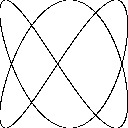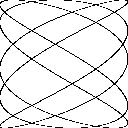#### Web Exercises

1. Word and line count. Modify WordCount.java so that reads in text from standard input and prints out the number of characters, words, and lines in the text.
2. Rainfall problem. Write a program Rainfall.java that reads in nonnegative integers (representing rainfall) one at a time until 999999 is entered, and then prints out the average of value (not including 999999).
3. Remove duplicates. Write a program Duplicates.java that reads in a sequence of integers and prints back out the integers, except that it removes repeated values if they appear consecutively. For example, if the input is 1 2 2 1 5 1 1 7 7 7 7 1 1, your program should print out 1 2 1 5 1 7 1.
4. Run length encoding. Write a program RunLengthEncoder.java that encodes a binary input using run length encoding. Write a program RunLengthDecoder.java that decodes a run length encoded message.
5. Head and tail. Write programs Head.java and Tail.java that take an integer command line input N and print out the first or last N lines of the given file. (Print the whole file if it consists of <= N lines of text.)
6. Print a random word. Read a list of N words from standard input, where N is unknown ahead of time, and print out one of the N words uniformly at random. Do not store the word list. Instead, use Knuth's method: when reading in the ith word, select it with probability 1/i to be the selected word, replacing the previous champion. Print out the word that survives after reading in all of the data.
7. Caesar cipher. Julius Caesar sent secret messages to Cicero using a scheme that is now known as a Caesar cipher. Each letter is replaced by the letter k positions ahead of it in the alphabet (and you wrap around if needed). The table below gives the Caesar cipher when k = 3.
 Original: A B C D E F G H I J K L M N O P Q R S T U V W X Y Z Caesar: D E F G H I J K L M N O P Q R S T U V W X Y Z A B C 

For example the message "VENI, VIDI, VICI" is converted to "YHQL, YLGL, YLFL". Write a program Caesar.java that takes a command-line argument k and applies a Caesar cipher with shift = k to a sequence of letters read from standard input. If a letter is not an uppercase letter, simply print it back out.

8. Caesar cipher decoding. How would you decode a message encrypted using a Caesar cipher? Hint: you should not need to write any more code.
9. Parity check. A Boolean matrix has the parity property when each row and each column has an even sum. This is a simple type of error-correcting code because if one bit is corrupted in transmission (bit is flipped from 0 to 1 or from 1 to 0) it can be detected and repaired. Here's a 4 x 4 input file which has the parity property:
 1 0 1 0 0 0 0 0 1 1 1 1 0 1 0 1 

Write a program ParityCheck.java that takes an integer N as a command line input and reads in an N-by-N Boolean matrix from standard input, and outputs if (i) the matrix has the parity property, or (ii) indicates which single corrupted bit (i, j) can be flipped to restore the parity property, or (iii) indicates that the matrix was corrupted (more than two bits would need to be changed to restore the parity property). Use as little internal storage as possible. Hint: you do not even have to store the matrix!

10. Takagi's function. Plot Takagi's function: everywhere continuous, nowhere differentiable.
11. Hitchhiker problem. You are interviewing N candidates for the sole position of American Idol. Every minute you get to see a new candidate, and you have one minute to decide whether or not to declare that person the American Idol. You may not change your mind once you finish interviewing the candidate. Suppose that you can immediately rate each candidate with a single real number between 0 and 1, but of course, you don't know the rating of the candidates not yet seen. Devise a strategy and write a program AmericanIdol that has at least a 25% chance of picking the best candidate (assuming the candidates arrive in random order), reading the 500 data values from standard input.

Solution: interview for N/2 minutes and record the rating of the best candidate seen so far. In the next N/2 minutes, pick the first candidate that has a higher rating than the recorded one. This yields at least a 25% chance since you will get the best candidate if the second best candidate arrives in the first N/2 minutes, and the best candidate arrives in the final N/2 minutes. This can be improved slightly to 1/e = 0.36788 by using essentially the same strategy, but switching over at time N/e.

12. Nested diamonds. Write a program Diamonds.java that takes a command line input N and plots N nested squares and diamonds. Below is the desired output for N = 3, 4, and 5.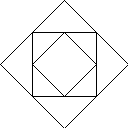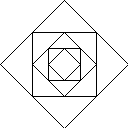13. Regular polygons. Create a function to plot an N-gon, centered on (x, y) of size length s. Use the function to draws nested polygons like the picture below.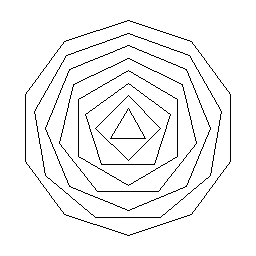14. Bulging squares. Write a program BulgingSquares.java that draws the following optical illusion from Akiyoshi Kitaoka The center appears to bulge outwards even though all squares are the same size.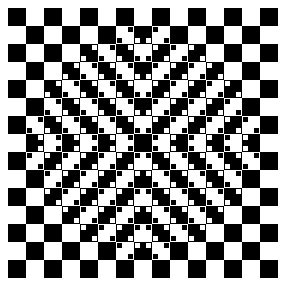15. Spiraling mice. Suppose that N mice that start on the vertices of a regular polygon with N sides, and they each head toward the nearest other mouse (in counterclockwise direction) until they all meet. Write a program to draw the logarithmic spiral paths that they trace out by drawing nested N-gons, rotated and shrunk as in this animation.
16. Spiral. Write a program to draw a spiral like the one below.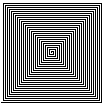17. Globe. Write a program Globe.java that takes a real command-line argument α and plots a globe-like pattern with parameter α. Plot the polar coordinates (r, θ) of the function f(θ) = cos(α × θ) for θ ranging from 0 to 7200 degrees. Below is the desired output for α = 0.8, 0.9, and 0.95.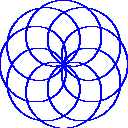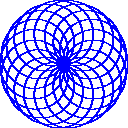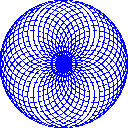18. Drawing strings. Write a program RandomText.java that takes a string s and an integer N as command line inputs, and writes the string N times at a random location, and in a random color.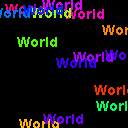19. 2D random walk. Write a program RandomWalk.java to simulate a 2D random walk and animate the results. Start at the center of a 2N-by-2N grid. The current location is displayed in blue; the trail in white.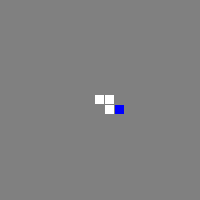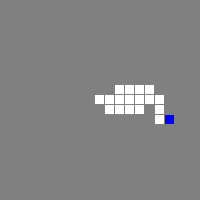20. Rotating table. You are seated at a rotating square table (like a lazy Susan), and there are four coins placed in the four corners of the table. Your goal is to flip the coins so that they are either all heads or all tails, at which point a bell rings to notify you that you are done. You may select any two of them, determine their orientation, and (optionally) flip either or both of them over. To make things challenging, you are blindfolded, and the table is spun after each time you select two coins. Write a program RotatingTable.java that initializes the coins to random orientations. Then, it prompts the user to select two positions (1-4), and identifies the orientation of each coin. Next, the user can specify which, if any of the two coins to flip. The process repeats until the user solves the puzzle.
21. Rotating table solver. Write another program RotatingTableSolver.java to solve the rotating table puzzle. One effective strategy is to choose two coins at random and flip them to heads. However, if you get really unlucky, this could take an arbitrary number of steps. Goal: devise a strategy that always solves the puzzle in at most 5 steps.

22. Hex. Hex is a two-player board game popularized by John Nash while a graduate student at Princeton University, and later commercialized by Parker Brothers. It is played on a hexagonal grid in the shape of an 11-by-11 diamond. Write a program Hex.java that draws the board.
23. Projectile motion with drag. Write a program BallisticMotion.java that plots the trajectory of a ball that is shot with velocity v at an angle theta. Account for gravitational and drag forces. Assume that the drag force is proportional to the square of the velocity. Using Newton's equations of motions and the Euler-Cromer method, update the position, velocity, and acceleration according to the following equations:
 v = sqrt(vx*vx + vy*vy) ax = - C * v * vx ay = -G - C * v * vy vx = vx + ax * dt vy = vy + ay * dt x = x + vx * dt y = y + vy * dt 

Use G = 9.8, C = 0.002, and set the initial velocity to 180 and the angle to 60 degrees.

24. Heart. Write a program Heart.java to draw a pink heart: Draw a diamond, then draw two circles to the upper left and upper right sides.25. Changing square. Write a program that draws a square and changes its color each second.
26. Simple harmonic motion. Repeat the previous exercise, but animate the Lissajous patterns as in this applet. Ex: A = B = wx = wy = 1, but at each time t draw 100 (or so) points with φx ranging from 0 to 720 degrees, and φx ranging from 0 to 1080 degrees.
27. Bresenham's line drawing algorithm. To plot a line segment from (x1, y1) to (x2, y2) on a monitor, say 1024-by-1024, you need to make a discrete approximation to the continuous line and determine exactly which pixels to turn on. Bresenham's line drawing algorithm is a clever solution that works when the slope is between 0 and 1 and x1 < x2.
 int dx = x2 - x1; int dy = y2 - y1; int y = y1; int eps = 0; for (int x = x1; x <= x2; x++) { StdDraw.point(x, y); eps += dy; if (2*eps >= dx) { y++; eps -= dx; } } 
28. Modify Bresenham's algorithm to handle arbitrary line segments.
29. Miller's madness. Write a program Madness.java to plot the parametric equation:
 x = sin(0.99 t) - 0.7 cos( 3.01 t) y = cos(1.01 t) + 0.1 sin(15.03 t) 
where the parameter t is in radians. You should get the following complex picture. Experiment by changing the parameters and produce original pictures.
30. Fay's butterfly. Write a program Butterfly.java to plot the polar equation:
 r = e^(cos t) - 2 cos(4t) + (sin(t/12)^5) 
where the parameter t is in radians. You should get an image like the following butterfly-like figure. Experiment by changing the parameters and produce original pictures.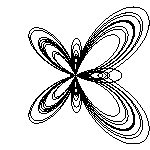31. Student database. The file students.txt contains a list of students enrolled in an introductory computer science class at Princeton. The first line contains an integer N that specifies the number of students in the database. Each of the next N lines consists of four pieces of information, separated by whitespace: first name, last name, email address, and section number. The program Students.java reads in the integer N and then N lines of data of standard input, stores the data in four parallel arrays (an integer array for the section number and string arrays for the other fields). Then, the program prints out a list of students in section 4 and 5.
32. Shuffling. In the October 7, 2003 California state runoff election for governor, there were 135 official candidates. To avoid the natural prejudice against candidates whose names appear at the end of the alphabet (Jon W. Zellhoefer), California election officials sought to order the candidates in random order. Write a program program Shuffle.java that takes a command-line argument N, reads in N strings from standard input, and prints them back out in shuffled order. (California decided to randomize the alphabet instead of shuffling the candidates. Using this strategy, not all N! possible outcomes are equally likely or even possible! For example, two candidates with very similar last names will always end up next to each other.)
33. Reverse. Write a program Reverse.java that reads in an arbitrary number of real values from standard input and prints them in reverse order.
34. Time series analysis. This problem investigates two methods for forecasting in time series analysis. Moving average or exponential smoothing.
35. Polar plots. Create any of these polar plots.
36. Java games. Use StdDraw.java to implement one of the games at javaunlimited.net.
37. Consider the following program.

 public class Mystery { public static void main(String[] args) { int N = Integer.parseInt(args); int[] a = new int[M]; while(!StdIn.isEmpty()) { int num = StdIn.readInt(); a[num]++; } for (int i = 0; i < M; i++) for (int j = 0; j < a[i]; j++) System.out.print(i + " "); System.out.println(); } } 

Suppose the file input.txt contains the following integers:

 8 8 3 5 1 7 0 9 2 6 9 7 4 0 5 3 9 3 7 6 

What is the contents of the array a after running the following command

 java Mystery 10 < input.txt 
38. High-low. Shuffle a deck of cards, and deal one to the player. Prompt the player to guess whether the next card is higher or lower than the current card. Repeat until player guesses it wrong. Game show: ???? used this.
39. Elastic collisions. Write a program CollidingBalls.java that takes a command-line argument n and plots the trajectories of n bouncing balls that bounce of the walls and each other according to the laws of elastic collisions. Assume all the balls have the same mass.
40. Elastic collisions with obstacles. Each ball should have its own mass. Put a large ball in the center with zero initial velocity. Brownian motion.
41. Statistical outliers. Modify Average.java to print out all the values that are larger than 1.5 standard deviations from the mean. You will need an array to store the values.
42. Optical illusions. Create a Kofka ring or one of the other optical illusions collected by Edward Adelson.
43. Computer animation. In 1995 James Gosling presented a demonstration of Java to Sun executives, illustrating its potential to deliver dynamic and interactive Web content. At the time, web pages were fixed and non-interactive. To demonstrate what the Web could be, Gosling presented applets to rotate 3D molecules, visualize sorting routines, and Duke cart-wheeling across the screen. Java was officially introduced in May 1995 and widely adopted in the technology sector. The Internet would never be the same.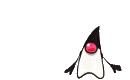Program Duke.java reads in the 17 images T1.gif through T17.gif and produces the animation. To execute on your computer, download the 17 GIF files and put in the same directory as Duke.java. (Alternatively, download and unzip the file duke.zip or duke.jar to extract all 17 GIFs.)
44. Cart-wheeling Duke. Modify Duke.java so that it cartwheels 5 times across the screen, from right to left, wrapping around when it hits the window boundary. Repeat this cart-wheeling cycle 100 times. Hint: after displaying a sequence of 17 frames, move 57 pixels to the left and repeat. Name your program MoreDuke.java.
45. Tac (cat backwards). Write a program Tac.java that reads lines of text from standard input and prints the lines out in reverse order.
46. Game. Implement the game dodge using StdDraw: move a blue disc within the unit square to touch a randomly placed green disc, while avoiding the moving red discs. After each touch, add a new moving red disc.
47. Simple harmonic motion. Create an animation like the one below from Wikipedia of simple harmonic motion.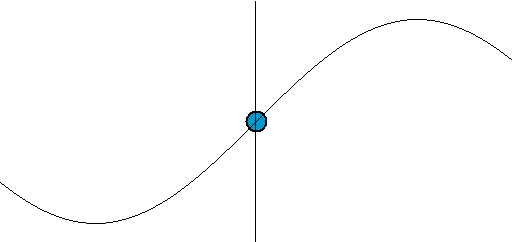48. Yin yang. Draw a yin yang using StdDraw.arc().
49. Twenty questions. Write a program QuestionsTwenty.java that plays 20 questions from the opposite point of view: the user thinks of a number between 1 and a million and the computer makes the guesses. Use binary search to ensure that the computer needs at most 20 guesses.
50. Write a program DeleteX.java that reads in text from standard input and deletes all occurrences of the letter X. To filter a file and remove all X's, run your program with the following command:
 % java DeleteX < input.txt > output.txt 
51. Write a program ThreeLargest.java that reads integers from standard input and prints out the three largest inputs.
52. Write a program Pnorm.java that takes a command-line argument p, reads in real numbers from standard input, and prints out their p-norm. The p-norm norm of a vector (x1, ..., xN) is defined to be the pth root of (|x1|p + |x2|p + ... + |xN|p).
53. Consider the following Java program.
 public class Mystery { public static void main(String[] args) { int i = StdIn.readInt(); int j = StdIn.readInt(); System.out.println((i-1)); System.out.println((j*i)); } } 

Suppose that the file input.txt contains

 5 1 
What does the following command do?
 java Mystery < input.txt 
54. Repeat the previous exercise but use the following command instead
 java Mystery < input.txt | java Mystery | java Mystery | java Mystery 
55. Consider the following Java program.
 public class Mystery { public static void main(String[] args) { int i = StdIn.readInt(); int j = StdIn.readInt(); int k = i + j; System.out.println(j); System.out.println(k); } } 

Suppose that the file input.txt contains the integers 1 and 1. What does the following command do?

 java Mystery < input.txt | java Mystery | java Mystery | java Mystery 
56. Consider the Java program Ruler.java.
 public class Ruler { public static void main(String[] args) { int n = StdIn.readInt(); String s = StdIn.readString(); System.out.println((n+1) + " " + s + (n+1) + s); } } 

Suppose that the file input.txt contains the integers 1 and 1. What does the following command do?

 java Ruler < input.txt | java Ruler | java Ruler | java Ruler 
57. Modify Add.java so that it re-asks the user to enter two positive integers if the user types in a non-positive integer.
58. Modify TwentyQuestions.java so that it re-asks the user to enter a response if the user types in something other than true or false. Hint: add a do-while loop within the main loop.
59. Nonagram. Write a program to plot a nonagram.
60. Star polygons. Write a program StarPolygon.java that takes two command line inputs p and q, and plots the {p/q}-star polygon.
61. Complete graph. Write a program to plot that takes an integer N, plots an N-gon, where each vertex lies on a circle of radius 256. Then draw a gray line connecting each pair of vertices.
62. Necker cube. Write a program NeckerCube.java to plot a Necker cube.
63. What happens if you move the StdDraw.clear(Color.BLACK) command to before the beginning of the while loop in BouncingBall.java? Answer: try it and observe a nice woven 3d pattern with the given starting velocity and position.
64. What happens if you change the parameter of StdDraw.show() to 0 or 1000 in BouncingBall.java?
65. Write a program to plot a circular ring of width 10 like the one below using two calls to StdDraw.filledCircle().
66. Write a program to plot a circular ring of width 10 like the one below using a nested for loop and many calls to StdDraw.point().
67. Write a program to plot the Olympic rings.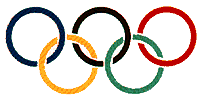68. Write a program BouncingBallDeluxe.java that embellishes BouncingBall.java by playing a sound effect upon collision with the wall using StdAudio and the sound file pipebang.wav.